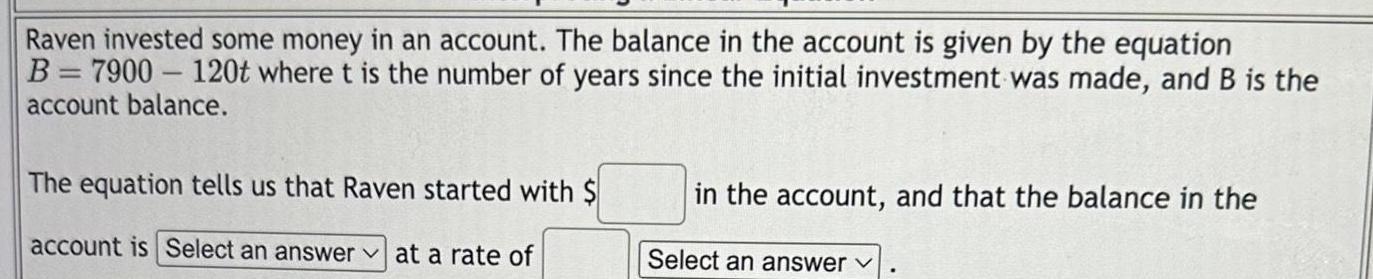Question:

# Raven invested some money in an account The balance in the

Last updated: 3/20/2023Raven invested some money in an account The balance in the account is given by the equation B 7900 120t where t is the number of years since the initial investment was made and B is the account balance The equation tells us that Raven started with account is Select an answer at a rate of in the account and that the balance in the Select an answer